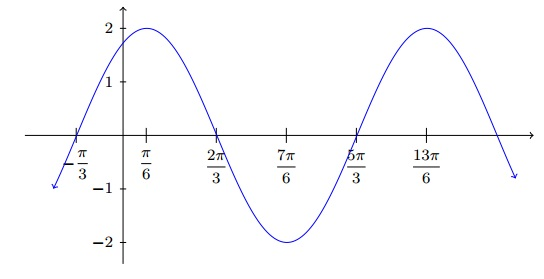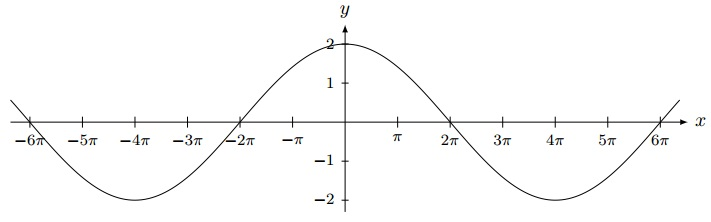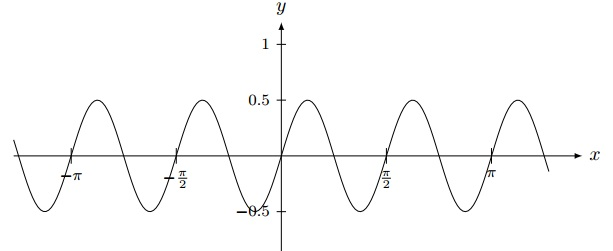# Questions on Graphs of Trigonometric Functions

Multiple choice questions on the properties of the graphs of trigonometric functions with answers at the bottom of the page.

### Question 1

What is the period of the graph shown below?a) π/3
b) 5 π/3
c) 2 π/3
d) 2 π

### Question 2

Which of the functions below represents the graph below?a) y = - cos(2x)
b) y = cos(2x)
c) y = - cos(x)
d) y = cos(x)

### Question 3

Which of the trigonometric functions correspond to the graph shown below?a) 2 sin(x / 4)
b) 2 cos(4x)
c) 2 cos(x / 4)
d) 2 sin(4x)

### Question 4

Which of the functions below correspond to the given graph?a) y = - sin(2πx)
b) y = sin(2πx)
c) y = cos(2πx)
d) y = - sin(x)

### Question 5

What is the period of the graph below?a) 1
b) 2
c) π
d) 2 π

### Question 6

Find an appropriate function for the following graph:a) y = 0.5 cos (4πx)
b) y = 0.5 sin (x/4)
c) y = 0.5 sin (4x)
d) y = 0.5 cos (4x)

### Question 7

Which of the functions below correspond to the given graph?a) y = sin(x / 2 - 1/4)
b) y = sin(πx / 2 - π/4)
c) y = cos(πx / 2 - π/4)
d) y = sin(πx / 2)

### Question 8

What is the period of the graph below?a) 5π/4
b) π/4
c) π
d) 2 π